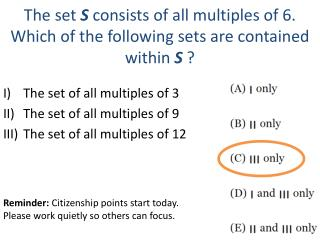DownloadDownload PresentationThe set  S consists of all multiples of 6. Which of the following sets are contained within S  ?

The set  S consists of all multiples of 6. Which of the following sets are contained within S  ?

Télécharger la présentationThe set  S consists of all multiples of 6. Which of the following sets are contained within S  ?

- - - - - - - - - - - - - - - - - - - - - - - - - - - E N D - - - - - - - - - - - - - - - - - - - - - - - - - - -
Presentation Transcript

1. The set Sconsists of all multiples of 6. Which of the following sets are contained within S ? • The set of all multiples of 3 • The set of all multiples of 9 • The set of all multiples of 12 Reminder: Citizenship points start today. Please work quietly so others can focus.

2. Parabolas y = ax2 + bx + c

3. Vertex (axis of symmetry, max/min) y-intercept x-intercept x-intercept

4. Properties of a Parabola

5. To Find the y-intercept: 1) Write the equation in standard form, set x equal to 0 and solve for y. 2) The y-intercept is alwaysc. y-int = ( 0,c)

6. To find the x-intercepts • Set y equal to 0 and solve for x. • The most common methods for solving quadratic equations are by factoring or using the quadratic formula.

7. Width = w Length = 5+w Area = 36 = l x w (5+w)(w) 5w + w2 = 36 The length of a rectangular window is 5 feet more than its width, w.  The area of the window is 36 square feet.  Write an equation that can be used to find the dimensions of the window.

8. Solve for w: 5w + w2 = 36 • Rearrange equation to set it equal to zero. • Factor to get: • In order for the product to equal 0, at least one of the factors has to be 0. • So you have • Therefore, • Which answer makes more sense and why? w2 + 5w - 36 = 0 (w + 9)(w - 4) = 0 W + 9 = 0 and w – 4 = 0 w=-9 and w=4 4 because dimensions cannot be negative.

9. Solving Using the Quadratic Formula Example: 5w + w2 = 36 → w2 + 5w - 36 = 0 For ax2 + bx + c = 0, the value of x is given by: Step 1: Note that Step 2: Substitute a = 1, b = 5, & c = -36

10. Step 3: SimplifyStep 4: Solve

11. Max/Min • If a is positive, the graph opens upward (smile) and you will have a min. • If a is negative, the graph opens downward (frown) and you will have a max. • The max/min is the y-coordinate of the vertex. y = ____ • The Axis of Symmetryis the x-coordinate of the vertex. x = _____

12. To find the Vertex… 1) Use to find the x- coordinate. 2) Plug the x-coordinate into the original equation and solve to find the y-coordinate.

13. Properties of Parabolas • x2 + 4x – 5 2) -x2 − 2x + 1